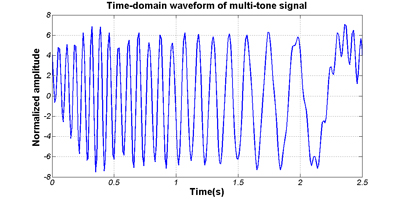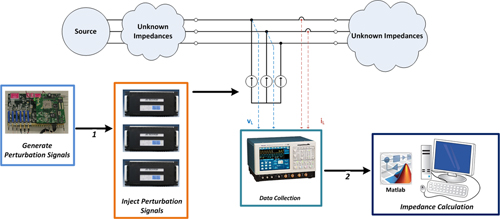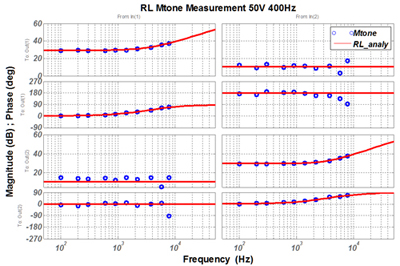Contact Us
LIBRARY

# Application of Multi-Tone Approach in Three-Phase AC System Impedance MeasurementFig. 1. (a) Multi-tone signal example.
AC and DC impedances of power converters are used for stability analysis of modern power systems at AC and DC interfaces. The basic idea for impedance extraction is an ac sweep approach with a sinusoidal signal injection, here denoted as single-tone. However, the approach is time consuming because the time-domain measurement will run many times. Therefore the approach of a multi-tone signal injection is implemented in which multiple frequency responses can be measured in only one time-domain measurement. A frequency selection algorithm is designed to assure the accuracy of measurement.

Three phase passive loads are measured and the result is compared with the analytical expression. The effectiveness of the multi-tone approach is verified.Fig. 1. (b)Fig. 2. Multi-tone measurement system setup.Fig. 3. Input impedance result comparison (line:analytical dot:multi-tone).
INDUSTRY PARTNERS